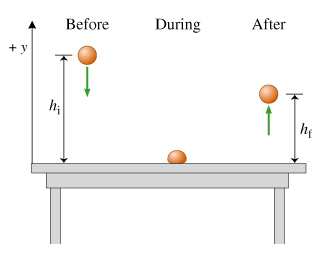# Problem: As shown in the figure (Figure 1) , a superball with mass m equal to 50 grams is dropped from a height of hi =1.5 m. It collides with a table, then bounces up to a height of hf = 1.0 m. The duration of the collision (the time during which the superball is in contact with the table) is tc = 15ms. In this problem, take the positive y direction to be upward, and use g = 9.8 m/s2 for the magnitude of the acceleration due to gravity. Neglect air resistance.a. Find the y component of the momentum, pbefore,y, of the ball immediately before the collision.b. Find the y component of the momentum of the ball immediately after the collision, that is, just as it is leaving the table.c. Find Jy, the y component of the impulse imparted to the ball during the collision.d. Find the y component of the time-averaged force Favg,y, in newtons, that the table exerts on the ball.e. Find Kafter - Kbefore, the change in the kinetic energy of the ball during the collision, in joules.

###### FREE Expert Solution

Law of conservation of energy:

$\overline{)\begin{array}{rcl}\mathbf{P}\mathbf{.}{\mathbf{E}}_{\mathbf{i}}\mathbf{+}\mathbf{K}\mathbf{.}{\mathbf{E}}_{\mathbf{i}}& {\mathbf{=}}& \mathbf{P}\mathbf{.}{\mathbf{E}}_{\mathbf{f}}\mathbf{+}\mathbf{K}\mathbf{.}{\mathbf{E}}_{\mathbf{f}}\\ \mathbf{m}\mathbf{g}{\mathbf{h}}_{\mathbf{i}}\mathbf{+}\frac{\mathbf{1}}{\mathbf{2}}\mathbf{m}{{\mathbf{v}}_{\mathbf{i}}}^{\mathbf{2}}& {\mathbf{=}}& \mathbf{m}\mathbf{g}{\mathbf{h}}_{\mathbf{f}}\mathbf{+}\frac{\mathbf{1}}{\mathbf{2}}\mathbf{m}{{\mathbf{v}}_{\mathbf{f}}}^{\mathbf{2}}\end{array}}$

Momentum:

$\overline{){\mathbf{p}}{\mathbf{=}}{\mathbf{m}}{\mathbf{v}}}$

Impulse:

$\overline{){\mathbf{J}}{\mathbf{=}}{\mathbf{∆}}{\mathbf{p}}}$

or

$\overline{){\mathbf{J}}{\mathbf{=}}{\mathbf{F}}{\mathbf{t}}}$

a.

From law of conservation of energy,

mghi + 0 = 0 + (1/2)mvi2

ghi = (1/2)vi2

vi = sqrt (2ghi) = sqrt[(2)(9.8)(1.5)] = 5.42 m/s

84% (152 ratings)###### Problem Details

As shown in the figure (Figure 1) , a superball with mass m equal to 50 grams is dropped from a height of hi =1.5 m. It collides with a table, then bounces up to a height of hf = 1.0 m. The duration of the collision (the time during which the superball is in contact with the table) is tc = 15ms. In this problem, take the positive y direction to be upward, and use = 9.8 m/s2 for the magnitude of the acceleration due to gravity. Neglect air resistance.a. Find the y component of the momentum, pbefore,y, of the ball immediately before the collision.

b. Find the y component of the momentum of the ball immediately after the collision, that is, just as it is leaving the table.

c. Find Jy, the y component of the impulse imparted to the ball during the collision.

d. Find the y component of the time-averaged force Favg,y, in newtons, that the table exerts on the ball.

e. Find KafterKbefore, the change in the kinetic energy of the ball during the collision, in joules.